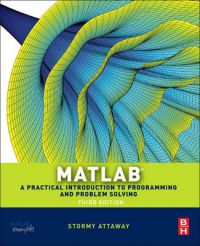Matlab

• 250,00 kr

Matlab: A Practical Introduction to Programming and Problem Solving. MATLAB has become the standard software tool for solving scientific and engineering problems due to its powerful built-in functions and its ability to program. Assuming no knowledge of programming, this book guides the reader through both programming and built-in functions to easily exploit MATLAB's extensive capabilities for tackling engineering problems. The book starts with programming concepts, such as variables, assignments, and selection statements, moves on to loops, and then solves problems using both the programming concept and the power of MATLAB. In-depth coverage is given to input/output, a topic fundamental to many engineering applications. The third edition of MATLAB: A Practical Introduction to Programming and Problem Solving has been updated to reflect the functionality of the current version of MATLAB. It features new and revised end-of-chapter exercises, stronger coverage of loops and vectorizing, and more engineering applications to help the reader learn this software tool in context. It presents programming concepts and MATLAB built-in functions side-by-side.It is a systematic, step-by-step approach, building on concepts throughout the book, facilitating easier learning. It includes sections on common pitfalls and programming guidelines direct students towards best practice.

The only book that gives a full introduction to programming in MATLAB combined with an explanation of MATLAB's powerful functions, enabling engineers to fully exploit MATLAB's power to solve engineering problems.
 Forfattere: Stormy Attaway Utgave: ukjent Språk: Engelsk Sidetall: 560 ISBN: 9780124058767 Vekt: 970 g Forlag: Butterworth-Heinemann Innbinding: Utgitt: 2013 Veil. pris: 0 kr